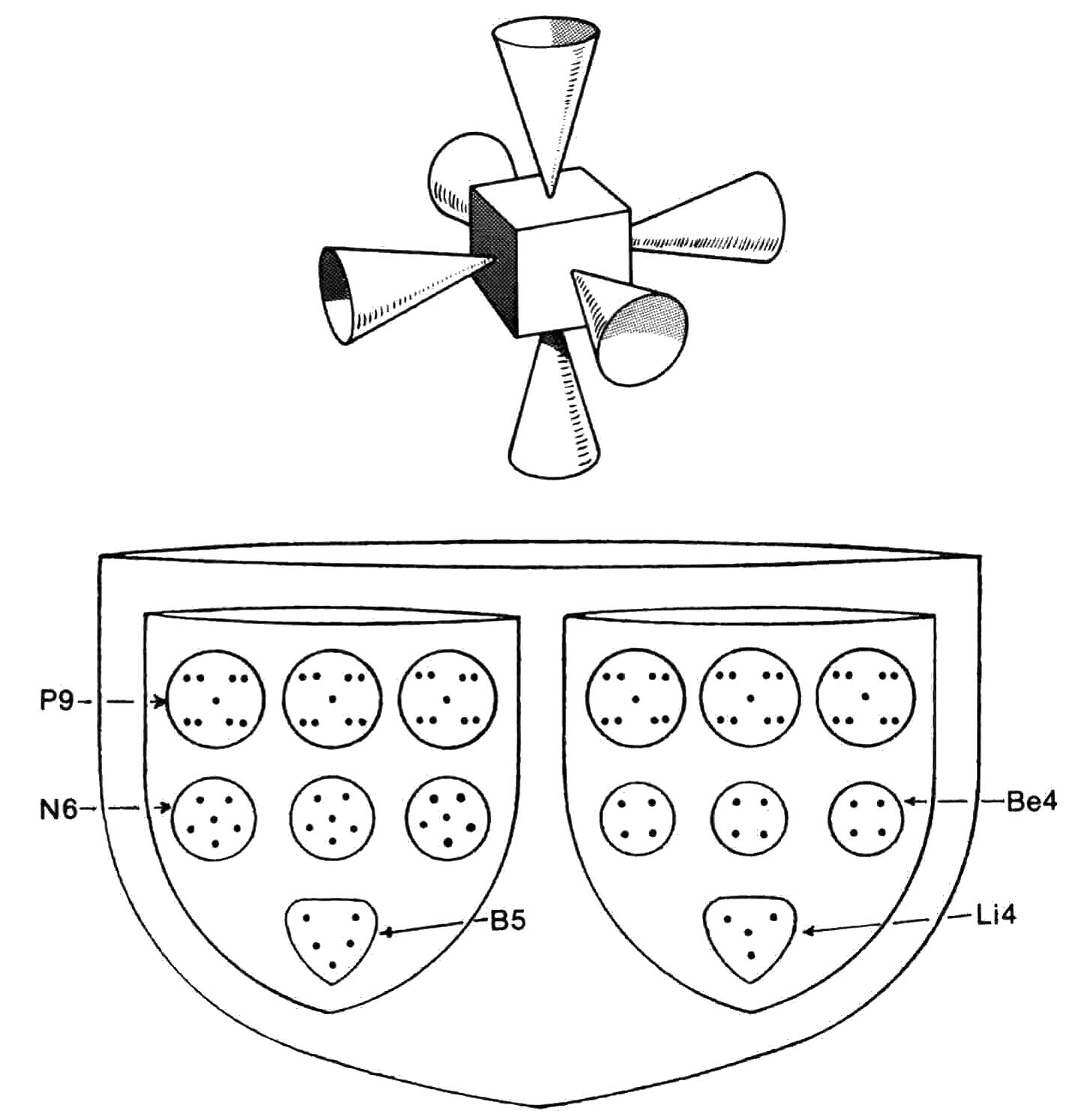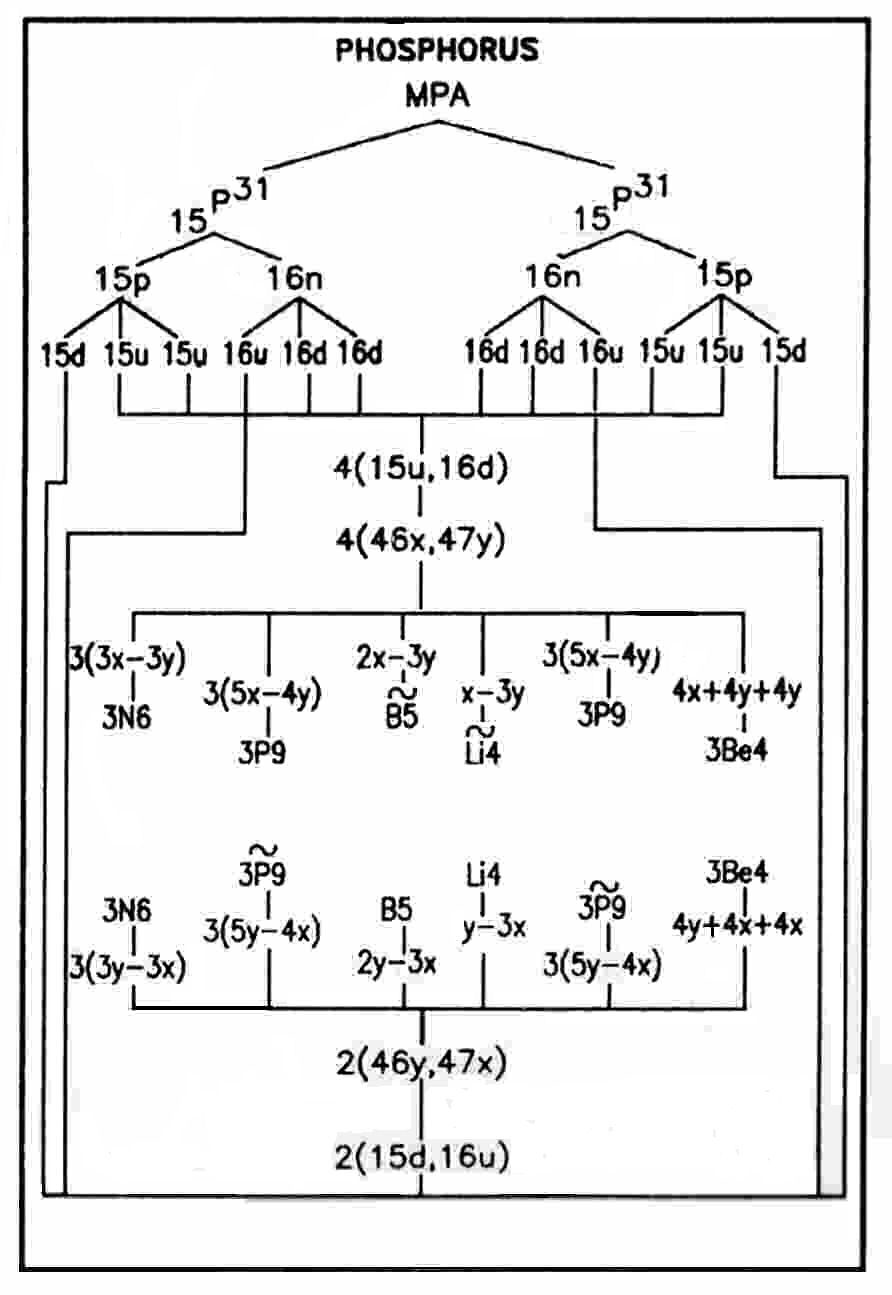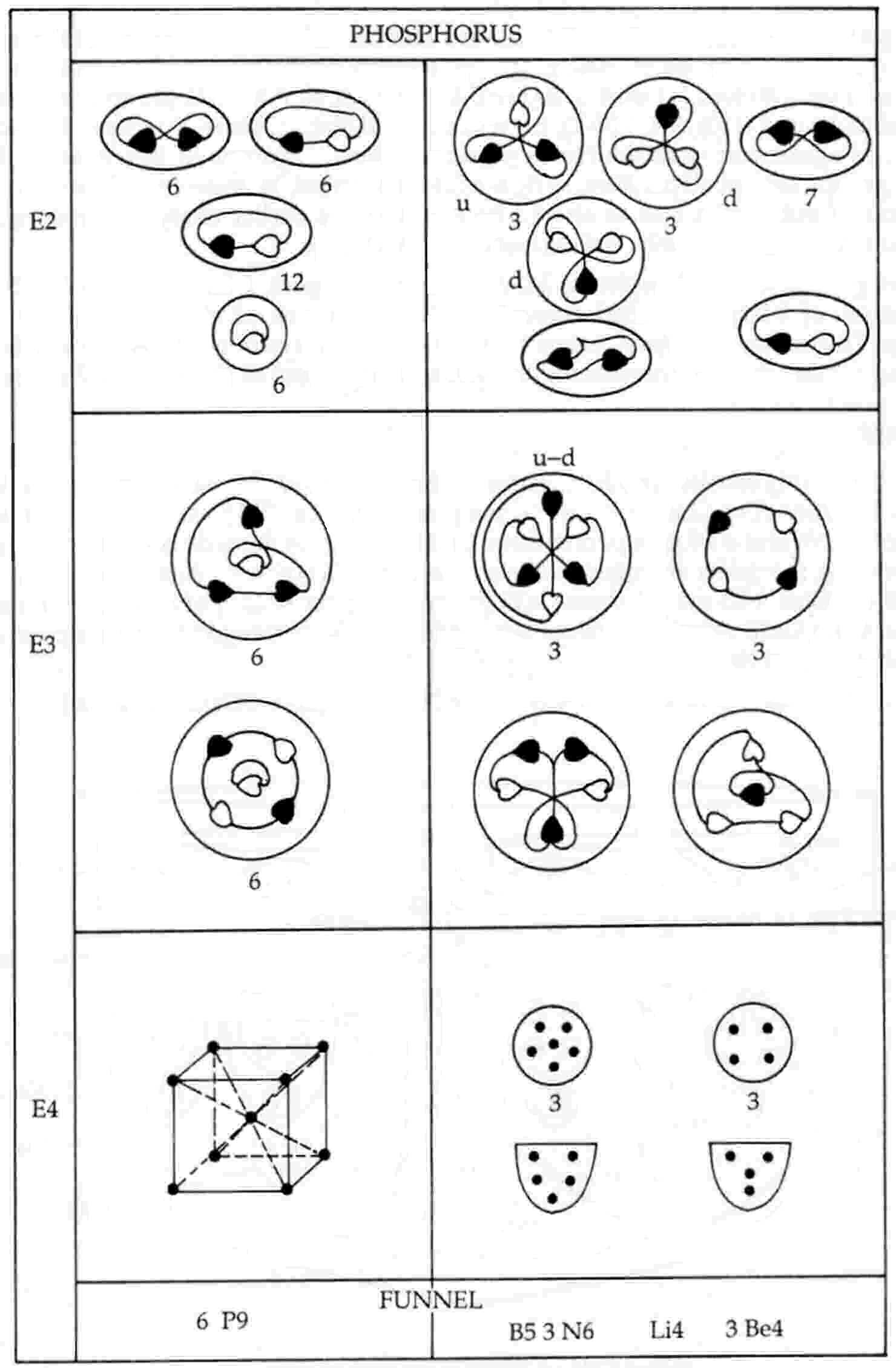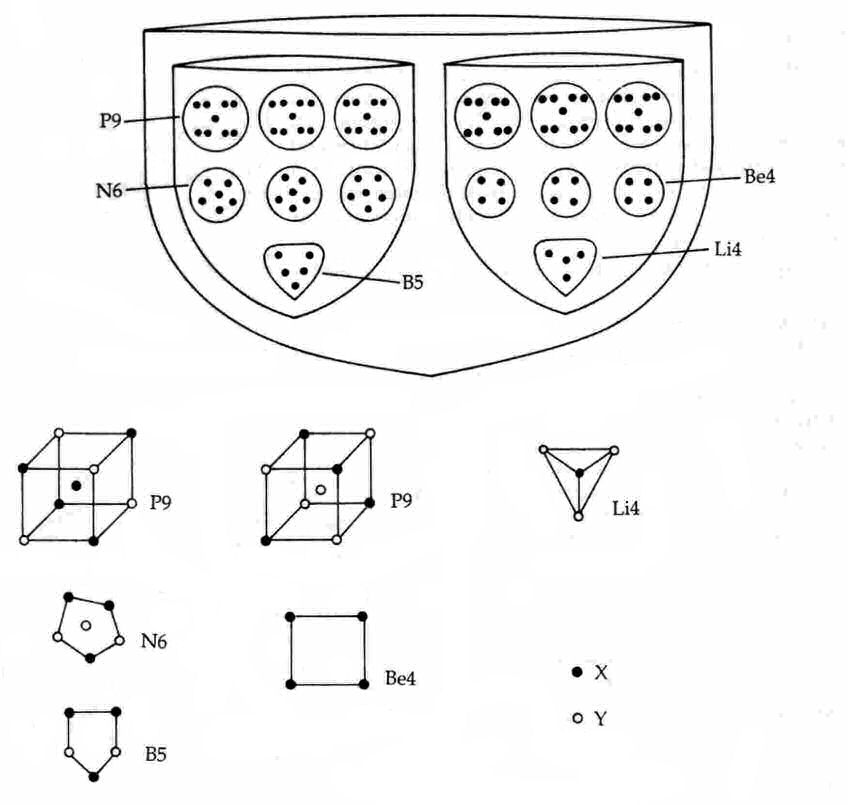The Phosphorus MPAThe phosphorus MPA is a face-centred cubic array of six funnels. Each funnel contains two segments. Segment A contains at the bottom a B5 group, then three N6 groups and, lastly, three spheres, each containing nine UPAs (P9) located at the centre and corners of a cube. Segment B contains a tetrahedral array of four UPAs (Li4), then three spheres containing four UPAs (Be4) and, finally, three spheres containing P9 groups.

Phosphorus MPA = 6[(B5 + 3N6 + 3P9) + (Li4 + 3Be4 + 3P9)].

The phosphorus MPA is formed from two P31 nuclei, which provide 558 subquarks — the same number as the number of UPAs. They comprise 278 X subquarks and 280 Y subquarks. As neither number is divisible exactly by 6, the funnels cannot have the samesubquark composition. Four funnels each contains the forty-six X subquarks and forty-seven Y subquarks making up fifteen u quarks and sixteen d quarks originally present in, respectively, the fifteen protons and sixteen neutrons in a P31 nucleus:

P9 (×6): 5X-4Y     Be4 (×3): 4X + 4Y + 4Y;
N6 (×3): 3X-3Y     B5′ (×1): 2X-3Y;
Li4′ (×1): X-3Y.

Two funnels each contains the forty-seven X subquarks and forty-six Y subquarks making up sixteen u quarks and fifteen d quarks originally present in, respectively, the sixteen neutrons and fifteen protons in a P31 nucleus:

P9′ (×6): 4X-5Y     Be4′ (×3): 4Y + 4X + 4X;
N6 (×3): 3Y-3X      B5 (×1): 2Y-3X;
Li4 (×1): Y-3X.

The particles in two funnels are the mirror states of their counterparts in the four other funnels. A P9 or P9′ is a body-centred cubic array of nine subquarks, consisting of intersecting tetrahedra of four X subquarks and four Y subquarks that are bound by strong forces to, respectively, an X or Y subquark acting as their nucleus. Repulsive Coulombic forces between the identical charges of the X subquarks create their tetrahedral orientation; similarly for the four Y subquarks. The disintegration diagram shown aboveSubquark composition of the particles in a funnel of the phosphorus MPA.

confirms the predicted composition of the P9 because it shows that it breaks up at stage E2 into a (+) duad (X-X), three (0) duads (X-Y) and a UPA:

5X-4Y → X-X + 3(X-Y) + Y.

The UPAs in an N6 released at the E3 stage rearrange themselves into an Ad6 group, which dissociates at the E2 stage into a (+) triplet (u quark) and a (−) triplet (d quark). It consists therefore of three X subquarks and three Y subquarks, in agreement with the prediction above. The B5 group breaks up at stage E2 into a (−) triplet (d quark) and a (+) duad (X-X), confirming its subquark composition:

2Y-3X → d quark (= 2Y-X) + X-X.

According to the disintegration diagram, the three Be4 groups and the Li4 group break up at stage E2 into seven (+) duads and a (0) duad. As, according to the disintegration diagrams of many MPAs, the (+) Be4 group breaks up into two (+) duads, the diagram for phosphorus implies that the Li4 group must consist of a (+) duad (X-X) and a (0) duad (X-Y), i.e., it is a bound state of three X subquarks and one Y subquark, in agreement with the above prediction for the groups in two funnels. But the three Be4 groups are not all positive, as the diagram indicates, because one of them is a bound state of four Y subquarks and so should have been depicted as negative. As earlier analyses have suggested, these groups were, presumably, not examined individually because they were assumed to be similar.

Home